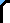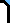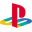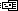Cheats DS GBA PC PS2 PS3 PSP Wii Xbox Xbox 360 New ReviewsSite Search

This Site WebTwisted MetalEmail this Page  |Printer Friendly Version

```Passwords.

Secret Battle:

Square Triangle Circle Square Square

Special Helicopter View:

Circle Circle Triangle X, (blank)

Minion Found:

Triangle X Circle Square Triangle

Invincibility:

Square Triangle X (space) Circle

Warehouse District:

Circle Triangle Square Circle Circle

Freeway Free For All:

X Square Square Circle Triangle

River Park Rumble:

X Triangle Square Circle Square

Assault on Cyburbia:

X Square Triangle Triangle Triangle

Rooftop Combat:

Square Triangle X Circle X
```